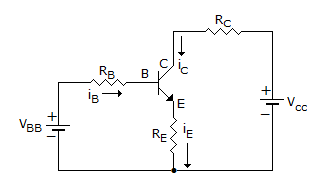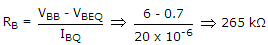# Electronics and Communication Engineering - Analog Electronics - Discussion

20.

The transistor of following figure in Si diode with a base current of 40 μA and ICBO = 0, if VBB = 6V, RE = 2 kΩ and β = 90, IBQ = 20 μA then RB =[A]. 200 kΩ [B]. 265 kΩ [C]. 150 kΩ [D]. 100 kΩ

Explanation:.

 Atul said: (Aug 21, 2014) What about Re?

 Balu said: (Feb 23, 2015) Why we not consider loop for i/p side?

 Arnab Bhattacharya said: (Jan 19, 2016) Ic = βIB + (β+1) ICBO => Ic = βIb [Since, ICBO = 0; given]. SO, Ic = 90*20 uA = 1.8 mA. Voltage drop across RE = IE*RE = (IC+IB)*RE = IC*RE (approx) = 1.8*2 = 3.6 V. Now, VBB = RB*IB + VBE + voltage across RE. 6 = RB*20 uA + 0.7 + 3.6 = RB*20 uA + 4.3. RB*20 uA = 1.7 => RB = 1.7/20 uA = 85 KOhm. So, my result is none of the above options. Please mention if I am missing something. Thanks in advance.

 Nitesh said: (Aug 15, 2016) I also agree with Arnab. Can anyone please clarify, if we are wrong somewhere.

 Raghu said: (Aug 17, 2016) What is 40 μA? Why it is given here?

 Bharadwaz said: (Nov 15, 2016) Yeah! I have the same doubt. Why shouldn't we consider input loop to find Rb? I don't think the procedure to find the Rb changes, if the operating point conditions are giving? If I am wrong, please correct me.

 Roni said: (Mar 23, 2017) What is the difference between IB AND IBQ? Please anyone tell me.

 Srujana said: (Mar 31, 2017) Ibq is base current at operating point taken as 0.7, this 0.7 is the barrier potential voltage at base.

 Pramit said: (Jul 10, 2017) The given solution is incorrect. We have to consider the drop across the emitter resistor. And we have to include it in the input loop equation.

 Vijay said: (Apr 16, 2018) Hi, I'm getting an answer between 100 and 150 whatever the answer is. The given answer is wrong we should consider the emitter resistor. Please, anyone, explain me.

 Artigo said: (Oct 10, 2018) ICBO = 0 implying IC = 0 => IE. So emitter voltage was not considered. Hence, the equation has only VBB - VRBQ - VBEQ = 0.